• 大整数乘法和我们小学学过的乘法公式一样（如下图），就是按位相乘，两个数中的每一位彼此相乘，然后将相同列的结果加起来，最后统一处理进位即可。#include <iostream> #include <cstring> using ...
大整数乘法和我们小学学过的乘法公式一样（如下图），就是按位相乘，两个数中的每一位彼此相乘，然后将相同列的结果加起来，最后统一处理进位即可。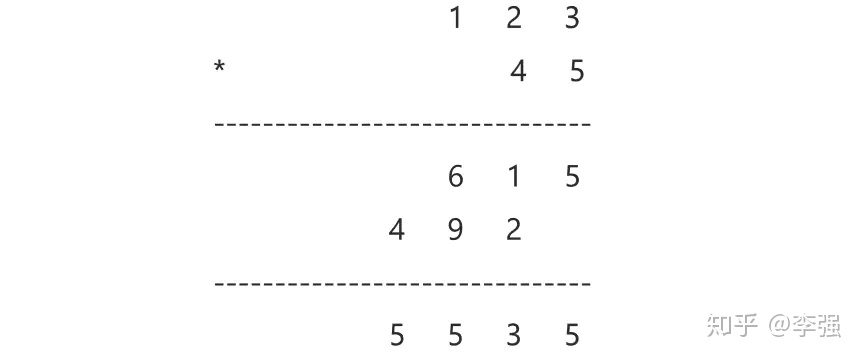#include <iostream>
#include <cstring>
using namespace std;
#define MAX_N 1000
​
void mul(int *num1, int *num2, int *res) { //乘法函数
res = num1 + num2; //使用num1, num2来存储数的位数，结果最多不超过num1 + num2位
for (int i = 1; i <= num1; i++) { //逐位相乘
for (int j = 1; j <= num2; j++) {
res[i + j] += num1[i] * num2[j];
}
}
for (int i = 1; i <= res; i++) {//处理进位
if (res[i] < 10) continue;
res[i + 1] += res[i] / 10;
res[i] %= 10;
i == res && res++;
}
}
​
int main() {
char str1[MAX_N + 5], str2[MAX_N + 5];
int num1[MAX_N + 5], num2[MAX_N +5], res[MAX_N + 5] = {0};  //注意res数组需要初始化，否则结果可能不正确
cin >> str1 >> str2;
num1 = strlen(str1);
//将逆序存储的字符串转化为顺序存储的整型
for (int i = 0; str1[i]; i++) {
num1[num1 - i] = str1[i] - '0';
}
num2 = strlen(str2);
for (int i = 0; str2[i]; i++) {
num2[num2 - i] = str2[i] - '0';
}
mul(num1, num2, res);
for (int i = res; i > 1; i--) { //第0位用来保存长度，第一位并没有使用
cout << res[i];
}
cout << endl;
return 0;
}以上就是大整数乘法的全部内容了。大家有什么问题，欢迎随时提问。
展开全文• 利用二分法和递归计算任意长度整数相乘以下复杂度分析有问题，在于 划分为 A12(n2)，这样才相当于移位；程序中采用string直接+'0'的方式来*10第一次的代码有漏洞，已更正我们可以把规模n变成n/2和n/2（把以1位为单位...
利用二分法和递归计算任意长度整数相乘以下复杂度分析有问题，在于 划分为 A12(n2)，这样才相当于移位；程序中采用string直接+'0'的方式来*10第一次的代码有漏洞，已更正我们可以把规模n变成n/2和n/2（把以1位为单位规模为n的问题 变成 以n/2为单位的规模为2的问题），把规模m变成m/2和m/2（把以1位为单位规模为m的问题 变成 以m/2为单位的规模为2的问题），如此，原来的大整数相乘就变成了两个2位数相乘，只不过低位向高位的进制不再是10，而是和。更一般地，我们把整数A由规模n分为n1和n2，把整数B由规模m分为m1和m2，如下图：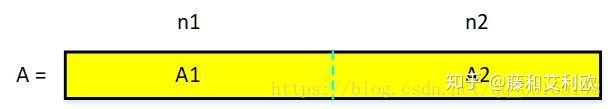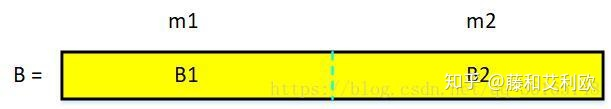则A分为n1位的A1和n2位，B分为m1位的B1和m2位的B2，如下式所示：以此类推，我们可以把A1、A2、B1、B2继续划分，直至最小单位。（这里在编程时需要用递归实现)于是注意用2^n做乘法相当于移位运算，需要O(n)时间。此公式中有4次乘法运算，3次加法运算，则故T(n)=O(n^2)而A1B1 A2B2已经计算过，故乘法运算次数减少为3次产生递推式// 四次乘法，3次加法
#include <iostream>
#include <string>
#include <sstream>

using namespace std;

string multi(string A, string B); //计算大整数相乘
string Plus(string q, string w, string e, string r); //计算大整数相加
stringstream ss;

int main() {
string A, B;
while (cin >> A >> B) {
cout << multi(A, B) << endl;
}
return 0;
}

string multi(string A, string B) {
int len_A = A.length();
int len_B = B.length();
if (len_A == 1) {
if (len_B == 1) { //最基本的情况：A和B都是一位数，把A、B从string转为int（我这里用的stringstream），然后相乘后转回为string型return回去。
ss << A;
int a;
ss >> a;
ss.clear();
ss << B;
int b;
ss >> b;
ss.clear();
ss << b*a;
string str_out;
ss >> str_out;
ss.clear();
return str_out;
}
else {//A是个位数，B不是的情况下，按照分治的思想把B分开分别与A相乘。
string B1, B2;
B1 = B.substr(0, len_B / 2);
B2 = B.substr(len_B / 2);
string AB1 = multi(A, B1);
string AB2 = multi(A, B2);
cout<<AB1<<endl<<AB2<<endl;
if (AB2.length() > B2.length()) {   //此时AB2最多比B2多出一位
string str = AB2.substr(0, 1);
/*ss << AB1;     漏洞,此时AB1可能已经超出int范围了
int ab1;
ss >> ab1;*/
string tp0(AB1.length()-1,'0');
str=tp0+str;
string u0(AB1.length(),'0');
AB1=Plus(AB1,str,u0,u0);
return AB1 + AB2.substr(1);
}
else
return AB1 + AB2;
}
}
else {
if (len_B == 1) {//B是个位数，A不是的情况与上述A是个位数B不是的情况相同。
string A1, A2;
A1 = A.substr(0, len_A / 2);
A2 = A.substr(len_A / 2);
string A1B = multi(A1, B);
string A2B = multi(A2, B);
if (A2B.length() > A2.length()) {
string str = A2B.substr(0, 1);
string tp0(A1B.length()-1,'0');
str=tp0+str;
string u0(A1B.length(),'0');
A1B=Plus(A1B,str,u0,u0);
return A1B + A2B.substr(1);
}
else {
return A1B + A2B;
}
}
else {//A和B都不是个位数，就按照上述方法分治就可以了，只是为了最后相加的时候方便，把返回的四个部分都用0凑成了位数相同的。
string A1, A2, B1, B2;
A1 = A.substr(0, len_A / 2);
A2 = A.substr(len_A / 2);
B1 = B.substr(0, len_B / 2);
B2 = B.substr(len_B / 2);
string part1_ = multi(A1, B1);
string part1_0(A2.length()+B2.length(), '0');   //长度为A2.length()+B2.length()的0串
part1_ = part1_ + part1_0;                      //A1B1*10(n2,m2)
string part2_ = multi(A2, B2);
string part2_00(part1_.length() - part2_.length(), '0');
part2_ = part2_00 + part2_;                     //A2B2，高位补0
string part3_ = multi(A1, B2);
string part3_0(A2.length(), '0');
part3_ = part3_ + part3_0;                    //A1B2*10(n2)
string part3_00(part1_.length() - part3_.length(), '0');
part3_ = part3_00 + part3_;
string part4_ = multi(A2, B1);
string part4_0(B2.length(), '0');
part4_ = part4_ + part4_0;
string part4_00(part1_.length() - part4_.length(), '0');
part4_ = part4_00 + part4_;
return Plus(part1_, part2_, part3_, part4_);   //未改进的第一种算法
}
}
}

string Plus(string q, string w, string e, string r) { //大整数相加，qwer位数相同
int len_q = q.length();
string y, out;
int a, b, c, d;
for (int i = 1; i <= len_q; i++) {  //逐位相加
ss << q.substr(len_q - i, 1);
ss >> a;
ss.clear();
ss << w.substr(len_q - i, 1);
ss >> b;
ss.clear();
ss << e.substr(len_q - i, 1);
ss >> c;
ss.clear();
ss << r.substr(len_q - i, 1);
ss >> d;
ss.clear();
ss << a + b + c + d;
ss >> y;
ss.clear();
if (i == 1)
out = y;
else if (out.length() > i - 1) {  //进一位
ss << out.substr(0, 1);
ss >> a;
ss.clear();
ss << y;
ss >> b;
ss.clear();
ss << a + b;
ss >> y;
ss.clear();
out = y + out.substr(1);
}
else {
out = y + out;
}
}
return out;
}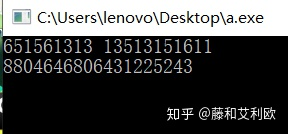// 三次乘法。由于还没有实现大整数减法（或支持负数的加法），暂时还是伪代码
#include <iostream>
#include <string>
#include <sstream>

using namespace std;

string multi(string A, string B); //计算大整数相乘
string Plus(string q, string w, string e, string r); //计算大整数相加
stringstream ss;

int main() {
string A, B;
while (cin >> A >> B) {
cout << multi(A, B) << endl;
}
return 0;
}

string multi(string A, string B) {
int len_A = A.length();
int len_B = B.length();
if (len_A == 1) {
if (len_B == 1) { //最基本的情况：A和B都是一位数，把A、B从string转为int（我这里用的stringstream），然后相乘后转回为string型return回去。
ss << A;
int a;
ss >> a;
ss.clear();
ss << B;
int b;
ss >> b;
ss.clear();
ss << b*a;
string str_out;
ss >> str_out;
ss.clear();
return str_out;
}
else {//A是个位数，B不是的情况下，按照分治的思想把B分开分别与A相乘。
string B1, B2;
B1 = B.substr(0, len_B / 2);
B2 = B.substr(len_B / 2);
string AB1 = multi(A, B1);
string AB2 = multi(A, B2);
cout<<AB1<<endl<<AB2<<endl;
if (AB2.length() > B2.length()) {   //此时AB2最多比B2多出一位
string str = AB2.substr(0, 1);
/*ss << AB1;     漏洞,此时AB1可能已经超出int范围了
int ab1;
ss >> ab1;*/
string tp0(AB1.length()-1,'0');
str=tp0+str;
string u0(AB1.length(),'0');
AB1=Plus(AB1,str,u0,u0);
return AB1 + AB2.substr(1);
}
else
return AB1 + AB2;
}
}
else {
if (len_B == 1) {//B是个位数，A不是的情况与上述A是个位数B不是的情况相同。
string A1, A2;
A1 = A.substr(0, len_A / 2);
A2 = A.substr(len_A / 2);
string A1B = multi(A1, B);
string A2B = multi(A2, B);
if (A2B.length() > A2.length()) {
string str = A2B.substr(0, 1);
string tp0(A1B.length()-1,'0');
str=tp0+str;
string u0(A1B.length(),'0');
A1B=Plus(A1B,str,u0,u0);
return A1B + A2B.substr(1);
}
else {
return A1B + A2B;
}
}
else {//A和B都不是个位数，就按照上述方法分治就可以了，只是为了最后相加的时候方便，把返回的四个部分都用0凑成了位数相同的。
string A1, A2, B1, B2;
A1 = A.substr(0, len_A / 2);
A2 = A.substr(len_A / 2);
B1 = B.substr(0, len_B / 2);
B2 = B.substr(len_B / 2);
string part1_ = multi(A1, B1);
part1_ = multi(2, part1_);
string part1_0(A2.length()+B2.length(), '0');   //长度为A2.length()+B2.length()的0串
part1_ = part1_ + part1_0;                      //2*A1B1*10(n2,m2)
string part2_ = multi(A2, B2);
part2_ =multi(2,part2_);
string part2_00(part1_.length() - part2_.length(), '0');
part2_ = part2_00 + part2_;                     //2*A2B2，高位补0

string part3_ = A1;
string part3_0(A2.length(), '0');             //(A1*10(n2)-A2)
part3_ = part3_ + part3_0;                    //A1*10(n2)  伪代码
part3_= part_3-A2                             //大整数减法（或支持负数的大整数加法），未实现

string part4_ = B1;                           //(B2-B1*10(m2))
string part4_0=(B2.length(), '0');
part4_ = part4_ + part4_0;
part4_= B2-part4_;

part5_= multi(part4_,part3_)

string part5_00(part1_.length() - part5_.length(), '0');
part5_ = part5_00 + part5_;
string u0(part1_.length(), '0');
return Plus(part1_, part2_, part5_, u0);
}
}
}

string Plus(string q, string w, string e, string r) { //大整数相加，qwer位数相同
int len_q = q.length();
string y, out;
int a, b, c, d;
for (int i = 1; i <= len_q; i++) {  //逐位相加
ss << q.substr(len_q - i, 1);
ss >> a;
ss.clear();
ss << w.substr(len_q - i, 1);
ss >> b;
ss.clear();
ss << e.substr(len_q - i, 1);
ss >> c;
ss.clear();
ss << r.substr(len_q - i, 1);
ss >> d;
ss.clear();
ss << a + b + c + d;
ss >> y;
ss.clear();
if (i == 1)
out = y;
else if (out.length() > i - 1) {  //进一位
ss << out.substr(0, 1);
ss >> a;
ss.clear();
ss << y;
ss >> b;
ss.clear();
ss << a + b;
ss >> y;
ss.clear();
out = y + out.substr(1);
}
else {
out = y + out;
}
}
return out;
}
参考 https://blog.csdn.net/qq_36165148/article/details/81132525​blog.csdn.net
展开全文• 大整数乘法是一个中低等难度的面试题：string multiply(const string& num1, const string& num2);为了简便起见，不存在前导空格、不存在负数（leetcode第43题）。今年招聘的时候用了很多次这个题目，基本上...
大整数乘法是一个中低等难度的面试题：string multiply(const string& num1, const string& num2);为了简便起见，不存在前导空格、不存在负数（leetcode第43题）。今年招聘的时候用了很多次这个题目，基本上连这个题目都写不出来的人极少（连这道题都写不出来的人肯定不会有下一题了）。大家的思路都是从右往左计算的。毕竟小学课本上的竖式都是从右往左计算的嘛。其实我一直想尝试一下从左往右计算，但是一直没动手写。今天整理面试题看到这道题，尝试写了一下，其实也挺简单，几分钟就写出来了。直接上代码吧。注释得比较清楚。// 在字符数串s的第index个字符上加上val，可能会产生进位
void add_char(string& s, int index, int val)
{
int tmp = s[index] - '0' + val;
s[index] = tmp % 10 + '0';
if(tmp / 10 > 0) // 进位，再加一次
add_char(s, index - 1, tmp / 10);
}

class Solution {
public:
string multiply(string num1, string num2) {
if(num1 == "0" || num2 == "0") return "0";

// result的位数最多是num1的长度加上num2的长度，或是len(num1) + len(num2) - 1
// 先按照最大长度分配内存，最后在去掉前导的0
string result(num1.size() + num2.size(), '0');

// 从左往右算
for(int i = 0; i < num1.size(); ++i) // 这里其实是乘数
{
for(int j = 0; j < num2.size(); ++j) // 这里是被乘数
{
int tmp = (num1[i] - '0') * (num2[j] - '0');
add_char(result, i + j + 1, tmp);
}
}

// 去掉前导的一个0
if(result == '0')
result.erase(0, 1);

return result;
}
};

展开全文• 采用数组实现的200位大整数乘法代码十分简洁，不到100行。对c语言学习很有帮助~
• C语言实现大整数乘法代码的完整代码及运行结果
﻿﻿
// Dmul.cpp : 定义控制台应用程序的入口点。
//
#include "stdafx.h"
#define MAXLENGTH 1000
#include <stdio.h>
#include <string.h>
void compute(char * a, char * b,char *c)
{
int i,j,m,n;
long sum,carry;
m = strlen(a)-1;
n = strlen(b)-1;
for(i=m;i>=0;i--)
a[i] -= '0';
for(i=n;i >=0;i--)
b[i] -='0';
c[m+n+2] ='\0';
carry =0;
for(i=m+n;i>=0;i--)
{
sum=carry;
if((j=(i-m))<0)
j=0;
for(;j<=i&& j <=n;j++)
sum += a[i-j]*b[j];
c[i+1] = sum %10 + '0'; /*算出保留的数字*/
carry = sum/10;
}
if((c=carry+'0')=='0') /* if no carry*/
c = '/040'; /* space */

}

void main()
{
char a[MAXLENGTH],b[MAXLENGTH],c[MAXLENGTH*2];
puts("*****         wmm        ******");
puts("*****     大整数乘法    ******");
puts("Input multiplier");
gets(a);
puts("Input multiplier");
gets(b);
compute(a,b,c);
puts("Answer:");
puts(c);
getchar();
}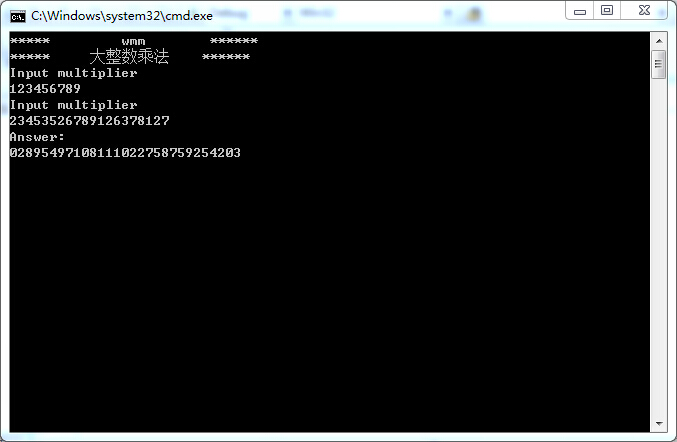展开全文应用 语言 控制台
• 可以实现c++大整数乘法，包含源代码，是c语言初学者的很好例子。
• 而在后来的C++语言中，C只是C++的一个子集，且C++中，已不推荐再用C的类库，但为了对已有代码的保护，还是对原来的头文件支持。 cstdio是c++从C的stdio.h继承来的，在前面加C同时不要H后缀，在C++环境当然是选用...
• 得到不是预期的结果，前几天在课堂上布置了一个求任意长度整数的阶乘，对于较小的数据，可以采用递归的算法即：f（n）=n*f（n-1）几行代码即可搞定，但是在整形的情况之下，则乘法的算法得重新实现。下面给出代码...
• C语言百位数的乘法运算 （不超过200位数） c语言中对于大数的加减乘除，需要解决的问题主要为大数的存储，c语言最大存储的正整数类型为long long int，远远小于我们所需要的百位数，这时就需要将数据的存储与数组...
• 代码使用方法是（以实例1为例）： 将该实例的源码，比如实例1的1.c文件（可以在001目录下找到）， 拷贝到tc编译器目录下，运行tc.exe，打开编译器， 按【F3】键或者“File->Open”菜单命令，打开1.c文件， ...
• 009 乘法口诀表 010 猜数字游戏 011 模拟ATM（自动柜员机）界面 012 用一维数组统计学生成绩 013 用二维数组实现矩阵转置 014 求解二维数组的最大/最小元素 015 利用数组求前n个质数 016 编制万年历 017 ...
• 003 求整数之积 004 比较实数大小 005 字符的输出 006 显示变量所占字节数 007 自增/自减运算 008 数列求和 009 乘法口诀表 010 猜数字游戏 011 模拟ATM（自动柜员机）界面 012 用一维数组统计学生成绩 ...
• 大整数加法代码，用C语言实现大整数的加法、减法、乘法、除法。算法
• 003 求整数之积 004 比较实数大小 005 字符的输出 006 显示变量所占字节数 007 自增/自减运算 008 数列求和 009 乘法口诀表 010 猜数字游戏 011 模拟ATM（自动柜员机）界面 012 用一维数组统计学生成绩 ...
• C程序源码.rar 001 第一个C程序 002 运行多个源文件 003 求整数之积 004 比较实数大小 005 字符的输出 006 显示变量所占字节数 007 自增/自减运算 008 数列求和 009 乘法口诀表 010 猜数字游戏
• 大整数.txt 字符串查找.txt 字符编辑.txt 字符编辑技术(插入和删除) .txt 完数.txt 定长串.txt 实例1.txt 实例2.txt 实例3.txt 小写数字转换成大写数字1.txt 小写数字转换成大写数字2.txt 小写数字转换...数据结构
• 大整数.txt 字符串查找.txt 字符编辑.txt 字符编辑技术(插入和删除) .txt 完数.txt 定长串.txt 实例1.txt 实例2.txt 实例3.txt 小写数字转换成大写数字1.txt 小写数字转换成大写数字2.txt 小写数字转换...
• C语言中，宽度最大的无符号整数类型是unsigned long long, 占8个字节。那么，如果整数超过8个字节，如何进行大数乘法呢？ 例如： \$ python Python 2.7.6 (default, Oct 26 2016, 20:32:47) ...<snip>....
• 代码使用方法是（以实例1为例）： 将该实例的源码，比如实例1的1.c文件（可以在001目录下找到）， 拷贝到tc编译器目录下，运行tc.exe，打开编译器， 按【F3】键或者“File->Open”菜单命令，打开1.c文件， 按...
• 大整数.c 完数.c 小孩分糖果.c 小明买书 平方根.c 数学算法 桃子猴问题 灯塔问题.c 百鸡百钱.c 简单计算器.c 苹果纠纷 递推.c 逻辑移动.c 阶乘递归.c 阿姆斯特朗数.c 黑白.c ./数学问题/凉东问题: 32.c re.c ...
• 003 求整数之积 004 比较实数大小 005 字符的输出 006 显示变量所占字节数 007 自增/自减运算 008 数列求和 009 乘法口诀表 010 猜数字游戏 011 模拟ATM（自动柜员机）界面 012 用一维数组统计学生成绩 ...
• 代码使用方法是（以实例1为例）： 将该实例的源码，比如实例1的1.c文件（可以在001目录下找到）， 拷贝到tc编译器目录下，运行tc.exe，打开编译器， 按【F3】键或者“File->Open”菜单命令，打开1.c文件， 按...
• 003 求整数之积 004 比较实数大小 005 字符的输出 006 显示变量所占字节数 007 自增/自减运算 008 数列求和 009 乘法口诀表 010 猜数字游戏 011 模拟ATM（自动柜员机）界面 012 用一维数组统计学生成绩 ...
• C语言实例解析精粹(第二版) 光盘代码 本文件包括以下内容： ※ 1、文件说明 ※ 2、源码操作说明 ※ 3、光盘目录清单 ◎ 源码操作说明 源代码使用方法是（以实例1为例）： 将该实例的源码，比如实例1的1.c文件（可以...
• C语言实例解析精粹(第二版) 电子书及源代码 附清晰版电子书及源代码 第一部分　基础篇 实例1　第一个C程序 实例2　运行多个源文件 实例3　求整数之积 实例4　比较实数大小 实例5　字符的输出 实例6　显示变量所占...
• C语言实例解析精粹 第一版 电子书及源代码 200 C 程序 第一部分 基础篇 001 第一个C程序 002 运行多个源文件 003 求整数之积 004 比较实数大小 005 字符的输出 006 显示变量所占字节数 007 自增/自减运算 ...
• 　书中以具体的实例为线索，特别注重对例题的分析、对知识点的归纳、对求解方法的引申，同时程序代码中融会了c语言的各种编程技巧，条理清晰，以方便读者举一反三，开发出符合特定要求的程序。本书的配套光盘中涵盖...
• 003 求整数之积 004 比较实数大小 005 字符的输出 006 显示变量所占字节数 007 自增/自减运算 008 数列求和 009 乘法口诀表 010 猜数字游戏 011 模拟ATM（自动柜员机）界面 012 用一维数组统计学生成绩 ...# 大整数乘法c语言代码c语言 订阅